### Sample Problem

In the figure below, a quarter-circle is inscribed in the big circle of radius 10. The top boundary curve of the shaded region is made by a circle with a center at the top of the shown circle. What is the area of the shaded region?#### SolutionIf we want to find the area of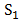, we should find the area offirst.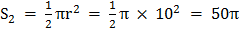.

We should use the following figures to find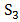.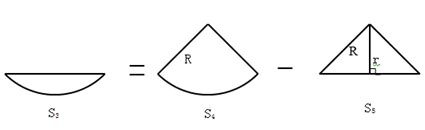.

To find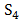, we should find R first. Since R is the base and height of the triangle,, and so.

The central angle of S4 = 90 because it is the angle measurement of 2 45o angles. Thus, since R2 = 200,.

Thus,And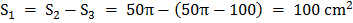.